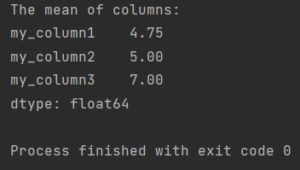Pandas How To Uncategorized How to calculate mean in Pandas

# How to calculate mean in Pandas

Here’s how to calculate mean in Pandas.## How to calculate mean in Pandas

To calculate a mean in Pandas just use a mean method which Pandas is offering to you. By default, it calculates the mean of all the columns in a dataframe, excluding missing values (represented as NaN in Pandas).

```import pandas as pd

my_df = pd.DataFrame({"my_column1": [9, 2, 3, 5],
"my_column2": [3, 7, 6, 4],
"my_column3": [4, 8, 8, 8]})

print(f'The mean of columns:\n{my_df.mean()}')

```
```The mean of columns:
my_column1    4.75
my_column2    5.00
my_column3    7.00
dtype: float64

```

You can also use the mean method to calculate the mean of a specific column by passing the name of the column as an argument. For example:

```import pandas as pd

my_df = pd.DataFrame({"my_column1": [9, 2, 3, 5],
"my_column2": [3, 7, 6, 4],
"my_column3": [4, 8, 8, 8]})

print(f'The mean of my_column1 column:\n{my_df['my_column1'].mean()}')

```

If there are missing values (represented as NaN) in the data, the mean calculation will exclude them by default. However, you can include them in the calculation by passing the argument skipna=False.

For more details see the documentation of mean function.

Tags:

## How to subtract dates in Pandas?How to subtract dates in Pandas?

In Pandas, you can subtract two dates to get the time delta between them. The result will be a Timedelta object, which represents the difference between two dates or times

## How to replace list of values with one valueHow to replace list of values with one value

In this post you will learn how to replace list of values with one value in Pandas.